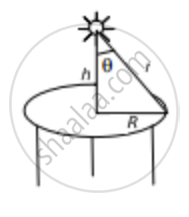Department of Pre-University Education, KarnatakaPUC Karnataka Science Class 12

# A Student is Studying a Book Placed Near the Edge of a Circular Table of Radius R. a Point Source of Light is Suspended Directly Above the Centre of the Table. - Physics

Sum

A student is studying a book placed near the edge of a circular table of radius R. A point source of light is suspended directly above the centre of the table. What should be the height of the source above the table so as to produce maximum illuminance at the position of the book?

#### SolutionLet the height of the source be h and the luminous intensity in the normal direction be I0.

So, illuminance on the book (E) is given by,

$E = \frac{I_0 \cos\theta}{r^2}$

From the figure we get

Cosθ = $\frac{h}{r}$

On substituting the value of Cosθ, we get

$E = \frac{I_0 h}{r^3}$

But r = $\sqrt{R^2 + h^2}$

$\therefore E=\frac{I_0 h}{\left( r^2 + h^2 \right)^\frac{3}{2}}$

For maximum illuminance,

$\frac{dE}{dH} = 0$

$\frac{dE}{dH} = \frac{I_0 \left[ \left( R^2 + h^2 \right)^\frac{3}{2} - \frac{3}{2}h \times \left( R^2 + h^2 \right)^\frac{1}{2} \times 2h \right]}{\left( R^2 + h^2 \right)^3} = 0$

$\Rightarrow \left( R^2 + h^2 \right)^\frac{1}{2} \left[ R^2 + h^2 - 3 h^2 \right] = 0$

$\Rightarrow R^2 - 2 h^2 = 0$

$\Rightarrow h = \frac{R}{\sqrt{2}}$

Concept: Light Process and Photometry
Is there an error in this question or solution?

#### APPEARS IN

HC Verma Class 11, Class 12 Concepts of Physics Vol. 1
Chapter 22 Photometry
Exercise | Q 13 | Page 455
Share